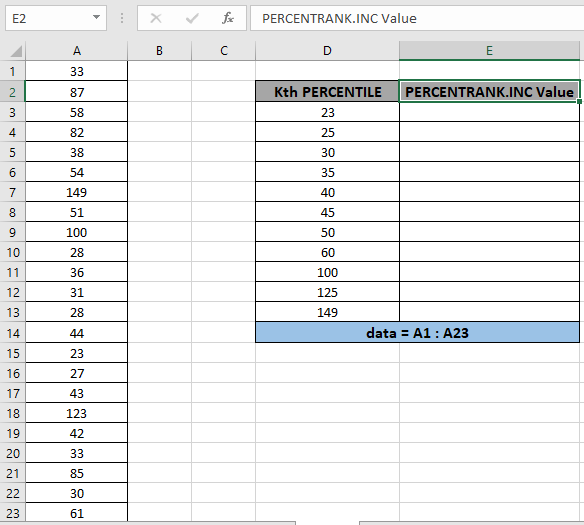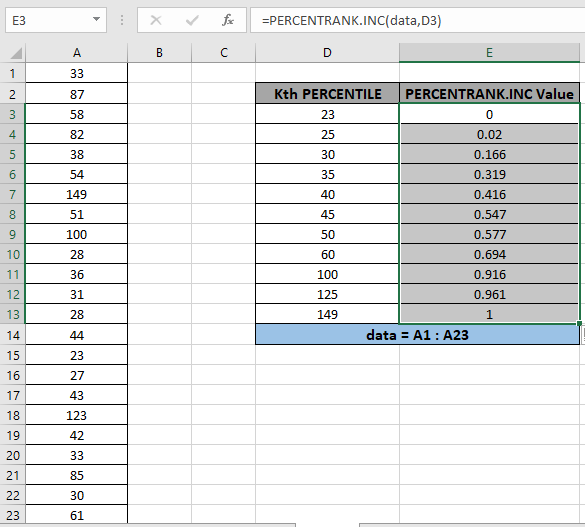# How to use the PERCENTRANK.INC Function

The PERCENTRANK.INC is a statistical function. In statistics, finding a percentage or percentile for the number on an array of numbers. The function checks the place of value on the list of values and returns its percentage place on the list of values.

PERCENTRANK.INC function takes the range of values as array and value. And returns the interpolate percentile for the given value.

Syntax:

 =PERCENTRANK.INC (array, x, [significance])

array : a list of numbers

x : number or value

[significance] : value upto decimal places. Default is 3

Example:

Let’s understand this function using it as an example.

Here we have a set of values as data ( A1 : A23 ) and we need to find some PERCENTRANK.INC values for the values. [significance] is set to default which is 3.Use the formula:

 = PERCENTRANK.INC ( data , D3)

data - array given using named range.

D3 - value to which percentile is calculated.

The function returns the percentage for the value ( 33 ) PERCENTRANK.INC value or minimum value for which the percentile is 0 .Now copy the formula to get different PERCENTRANK.INC values of the range to the remaining cells and formula as shown in the snapshot below.As you can see the results in the snapshot above.

Here are some of the observations gathered using the PERCENTRANK.INC function.

Note:

1. The function returns #VALUE! Error if the argument to the function is non numeric.
2. The function returns #NA! error if the value of x is less than the minimum value of the array or greater than the maximum value of the array.
3. The function returns #NUM! error if the value of significance is less than 1.
4. Significance value 1 returns 1 integer digit result like ( 0 ) but for the value 2 it returns the value upto 2 decimal places like (0.02)
5. The array argument to the function ignores text, Logic values or Date & Time values.

Hope you understood How to use PERCENTRANK.INC function in Excel. Explore more articles on statistical function like SMALL function & LARGE function here. Please state your query in the comment box below.

Related Articles

How to use the QUARTILE function in Excel

How to use the LARGE function in Excel

How to use the SMALL function in Excel

How to use Wildcards in Excel

Popular Articles

Edit a dropdown list

If with conditional formatting

If with wildcards

Vlookup by date

Terms and Conditions of use

The applications/code on this site are distributed as is and without warranties or liability. In no event shall the owner of the copyrights, or the authors of the applications/code be liable for any loss of profit, any problems or any damage resulting from the use or evaluation of the applications/code.